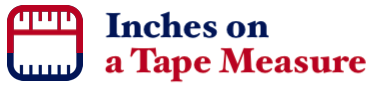Inches on a Tape MeasureHere you can locate and see inch measurements on a tape measure. In other words, we will illustrate on an image of a tape measure exactly where your inch measurement is. The inch measurements can be decimal numbers, fractions, and mixed numbers on a tape measure.

We made this tool so you can accurately pinpoint where any inch measurement is on a tape measure. It may be important to getting your project done correctly.

Numbers
For example, 50 or 1.23. Our tape measure is 100 inches long and will round your number if you enter too many decimals.

Where is inches on a tape measure?

Fractions
For example, 1/2 or 3/16. The numerator and denominator can range from 0 to 99.

Where is / inches on a ruler?

Mixed Numbers
For example, 1 1/3 or 2 1/5. The whole number can be max 20, the numerator must be less than the denominator, and the denominator must be 64 or less.

Where is   / inches on a ruler?

Here are some examples of what this tool can do for you:

3/4 inches on a tape measure

3/16 inches on a tape measure

3/8 inches on a tape measure

1 1/8 inches on a tape measure

1/6 inches on a tape measure

0.4 inches on a tape measure

1.75 inches on a tape measure

3 9/16 inches on a tape measure

1.25 inches on a tape measure